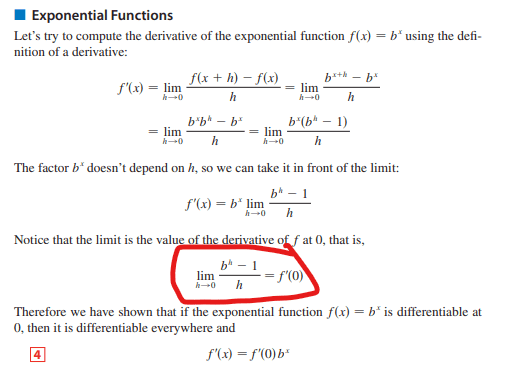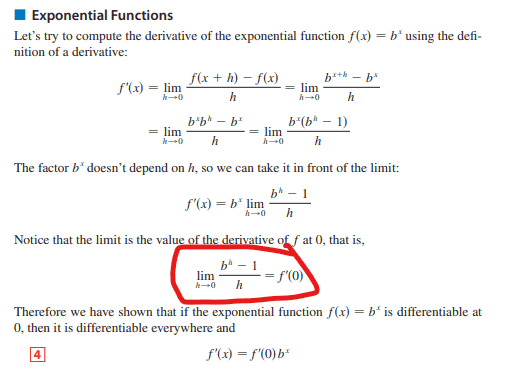# Computing the derivative of an exponential function

• ChiralSuperfields

#### ChiralSuperfields

Homework Statement
I am trying to understand why as h approach's zero ##\frac{b^h - 1}{h} = f'(0)##. Dose anybody please know of a good way to explain this? Many thanks!
Relevant Equations
Pls see belowThat is the definition of the derivative of ##f(x) = b^x## at ##x=0##. Notice that they do not say there that the limit and the derivative exist. In fact, the last sentence says "if the exponential function ##f(x) = b^x## is differentiable" (emphasis mine). So there is still something to prove.

•ChiralSuperfields
That is the definition of the derivative of ##f(x) = b^x## at ##x=0##. Notice that they do not say there that the limit and the derivative exist. In fact, the last sentence says "if the exponential function ##f(x) = b^x## is differentiable" (emphasis mine). So there is still something to prove.

How dose ##\lim_{x \rightarrow h} {\frac {b^h - 1} {h}} = f'(0)##?

Many thanks!

How dose ##\lim_{x \rightarrow h} {\frac {b^h - 1} {h}} = f'(0)##?
You have formula wrong. I think that ##\lim_{h \rightarrow 0} {\frac {f( x+h) - f(x)}{h}} = f'(x)## is a common definition of the derivative. Is that your definition of the derivative? Now plug in ##x=0##.

•ChiralSuperfields
Homework Statement:: I am trying to understand why as h approach's zero ##\frac{b^h - 1}{h} = f'(0)##. Dose anybody please know of a good way to explain this? Many thanks!
Relevant Equations:: Pls see belowFirst: The word is "Please", not Pls .

Also, you've been misspelling the word "Does". It is not "Dose".

So, you're back to posting a very detailed explanation of some math or physics topic, then asking some question regarding a detail which has been very well explained.

The best answer I can give as to why ##\displaystyle f'(0)=\lim_{h\to 0} \dfrac{b^h - 1}{h} ## is because ##\displaystyle b^0 = 1## .

•malawi_glenn, ChiralSuperfields and Mark44
You have formula wrong. I think that ##\lim_{h \rightarrow 0} {\frac {f( x+h) - f(x)}{h}} = f'(x)## is a common definition of the derivative. Is that your definition of the derivative? Now plug in ##x=0##.

If I plug in x = 0, to the definition of derivative (the expression that you mentioned, I get

##\frac{0}{0} = undefined ##. I am not sure where to go from here?

Many thanks!

•malawi_glenn
First: The word is "Please", not Pls .

Also, you've been misspelling the word "Does". It is not "Dose".

So, you're back to posting a very detailed explanation of some math or physics topic, then asking some question regarding a detail which has been very well explained.

The best answer I can give as to why ##\displaystyle f'(0)=\lim_{h\to 0} \dfrac{b^h - 1}{h} ## is because ##\displaystyle b^0 = 1## .

But plugging in h = 0 gives ##\frac{0}{0} = undefined##. How can ##f'(0)## be undefined since it is not a number?

Many thanks!

•malawi_glenn

If I plug in x = 0, to the definition of derivative (the expression that you mentioned, I get

##\frac{0}{0} = undefined ##. I am not sure where to go from here?

Many thanks!
Actually, you went too far. You asked this:
Homework Statement:: I am trying to understand why as h approach's zero ##\frac{b^h - 1}{h} = f'(0)##.
Plug in ##x=0## to get the definition ##f'(0) =\lim_{h \rightarrow 0} \frac{b^{0+h} - b^0}{h} = \lim_{h \rightarrow 0}\frac {b^h - 1}{h}##.
Notice that this is the definition, it does not say that the limits actually exist or the value of the limit. You are trying to prove that, which is a step too far for now. I assume that is proven somewhere else.

Last edited:
•ChiralSuperfields and malawi_glenn

But plugging in h = 0 gives ##\frac{0}{0} = undefined##. How can ##f'(0)## be undefined since it is not a number?

Many thanks!
That's not how to evaluate this limit.

You are reading something into what is stated which is not there.

•ChiralSuperfields
h should not be zero, should only be close to zero.

Try this, set b =2.72 and evalute that fraction at h = 0.001 and for h = 10^-6 and for h = 10^-9

You should see a pattern now.

Repeat the above but for b = 2.7183

Wha do you find?

Last edited:
•ChiralSuperfields